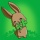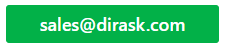Home
IT Knowledge
IT Career
Inspiration
Languages
EN

# JavaScript - how to use Monte Carlo method to calculate surface area (definite integral)?

8 points
Created by:JustMike
26120

Using JavaScript it is possible to calculate surface area (definite integral) in following way.

## 1. Circle surface area calculation example

Main idea of this method is to:

1. random big amount of points
2. check all time if every next one point is inside circle or not,
3. compute coefficinet by dividing number of points inside of circle by outsie one points
4. multiply the coefficient by square surface area - this way from proportion it is posible to get aproximated circle surface area.

Note: the longer the simulation is performed, the results are more precised.

``````// ONLINE-RUNNER:browser;

<!doctype html>
<html>
<style>

#my-canvas { border: 1px solid gray; }

</style>
<script>

function randomizePoint(range) {
return range * (Math.random() - 0.5);
}

// This method checks the point is inside circle
// with center at (0, 0) point.
//
var pointRadius = Math.sqrt(pointX * pointX + pointY * pointY);

return true;
}

return false;
}

window.Drawer = function(canvas) {
var context = canvas.getContext('2d');

var xCenter = canvas.width / 2;
var yCenter = canvas.height / 2;

this.clearCanvas = function() {
context.clearRect(0, 0, canvas.width, canvas.height);
};

this.drawPoint = function(x, y, color) {

var tmpX = Math.round(xCenter + x - 1);
var tmpY = Math.round(yCenter + y - 1);

context.strokeStyle = '#fff';
context.fillStyle = color || '#000';

context.fillRect(tmpX, tmpY, 2, 2);
};

this.drawCircle = function(x, y, radius) {

var tmpX = Math.round(xCenter + x - 1);
var tmpY = Math.round(yCenter + y - 1);

context.lineWidth = 0.5;
context.strokeStyle = '#ff0000';
context.fillStyle = '#fff';

context.beginPath();
context.arc(tmpX, tmpY, radius, 0, 2 * Math.PI);
context.stroke();
};
};

</script>
<body>
<canvas id="my-canvas" width="300" height="300"></canvas>
<script>

var canvas = document.querySelector('#my-canvas');

var drawer = new Drawer(canvas);

var squareArea = canvas.width * canvas.height;

var squarePointsCount = 0;
var circlePointsCount = 0;

drawer.clearCanvas();

function makeIteration() {

for(var i = 0; i < 100; ++i) {

// random coordinates from range -150 to +150
var pointX = randomizePoint(canvas.width);
var pointY = randomizePoint(canvas.height);

drawer.drawPoint(pointX, pointY, 'red');

circlePointsCount += 1;
} else {
drawer.drawPoint(pointX, pointY, 'silver');
}

squarePointsCount += 1;
}

// next iterations gives computation better precision
// according to number of randomized points

var randomPointsProportion = circlePointsCount / squarePointsCount;

var computedCircleArea = randomPointsProportion * squareArea;

var circleAreaError = Math.abs(expectedCircleArea - computedCircleArea);

console.clear();

console.log('Square surface area:');
console.log(' ' + squareArea + ' units^2');

console.log('Circle surface area:');
console.log(' Expected: ' + expectedCircleArea.toFixed(2) + ' units^2');
console.log(' Computed: ' + computedCircleArea.toFixed(2) + ' units^2');
console.log(' Error: ' + circleAreaError.toFixed(6) + ' units^2');
}

setInterval(makeIteration, 100);

</script>
</body>
</html>``````

## References

1. Monte Carlo method - Wikipedia